# Cosmology

### The Grand Unified Theory of Classical Physics – Collective Phenomena, High Energy Physics & Cosmology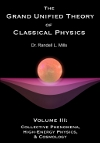View the Collective Phenomena… Slideshow (PDF)
View the book: Volume 3: Collective Phenomena…

View Related Papers: Classical Quantum Mechanics – R. Mills, Physics Essays, Vol. 16, No. 4, December (2003), pp. 433-498.

View Discussions of Canonical Experiments:
Electron Double-Slit Experiment
Aspect Experiment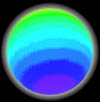The Neutron Charge Density Function
Shown in its inertial frame with positive and negative charge-density proportional to red intensity and blue intensity, respectively. The neutron is comprised of a linear combination of three orthogonal quarks, up, down, and down, of charge +2/3 e, -1/3 e, and -1/3 e, respectively, that form a two-dimensional spherical shell of charge having a radius of the Compton wavelength of the neutron. The quarks have the properties of an energy-to-matter transition state and spin about the z-axis with the centrifugal force of each quark balanced by the electric field of its gluon, a heavy photon, that is phase-locked to the spinning quark and inseparable from it.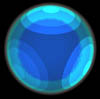The Neutron Mass Density Function
The neutron mass-density function in its inertial frame shown with the low and high mass-density proportional to red intensity and blue intensity, respectively. The neutron is comprised of a linear combination of three orthogonal quarks, up, down, and down, of equal mass, (1/3)*(Mn/(2*Pi)), that form a two-dimensional spherical shell of mass having a radius of the Compton wavelength of the neutron. The quarks which have the properties of an energy-to-matter transition state and spin about the z-axis with the centrifugal force of each quark balanced by the electric field of its gluon, a heavy photon, each of mass Mn(1-1/(2*Pi)), that is phase-locked to the spinning quark and inseparable from it. The brightness corresponds to the intensity of the two-dimensional radial gluon field.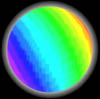The Proton Charge Density Function
Shown in its inertial frame with positive and negative charge-density proportional to red intensity and blue intensity, respectively. The proton is comprised of a linear combination of three orthogonal quarks, up, up, and down, of charge +2/3 e, +2/3 e, and -1/3 e, respectively, that form a two-dimensional spherical shell of charge having a radius of the Compton wavelength of the proton. The quarks have the properties of an energy-to-matter transition state and spin about the z-axis with the centrifugal force of each quark balanced by the electric field of its gluon, a heavy photon, that is phase-locked to the spinning quark and inseparable from it.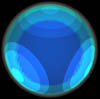The Proton Mass Density Function
Shown in its inertial frame with the low and high mass-density proportional to red intensity and blue intensity, respectively. The proton is comprised of a linear combination of three orthogonal quarks, up, up, and down, of equal mass, (1/3)*(Mp/(2*Pi)), that form a two-dimensional spherical shell of mass having a radius of the Compton wavelength of the proton. The quarks have the properties of an energy-to-matter transition state and spin about the z-axis with the centrifugal force of each quark balanced by the electric field of its gluon, a heavy photon, each of mass Mp(1-1/(2*Pi)), that is phase-locked to the spinning quark and inseparable from it. The brightness corresponds to the intensity of the two-dimensional radial gluon field.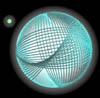Simulation of Pair Production
A linearly polarized photon of energy 1.02 MeV comprising the superposition of two oppositely circularly-polarized photons collides with a third body such as a proton. The photon transforms into a transition state intermediate between matter and energy, then forms a two-dimensional spherical shell with twice the mass of the electron and with the same radius as the photon, the electron Compton-wavelength bar. The shell comprises the superposition of the positron and the electron of opposite charges and each having h-bar of total angular momentum. This transition state ionizes, and the free particles propagate in different directions with linear momentum conserved.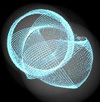Formation and Annihilation of Positronium
A free positron and electron are mutually attracted by the Coulombic force. A positron and an electron form a bound state called positronium that exists as a two-dimensional spherical shell of twice the mass of the electron with a radius of twice the Bohr radius. The particle provides the central force for the antiparticle. The shell comprises the superposition of the positron and the electron of opposite charges and each having h-bar of total angular momentum. Transitions between ortho and para magnetic states may occur. The pair transforms into a transition state intermediate between matter and energy. The annihilation is complete as two oppositely circularly-polarized photons each of 510 keV and having a radius of twice the electron Compton-wavelength bar (not to scale) propagate in opposite directions.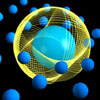Free Electrons in Superfluid Helium
Free electrons may be trapped as hollow bubbles in superfluid helium. The surrounding helium atoms maintain the bubble through Van der Waals forces at a fixed initial radius. The bubble may act as a resonator cavity to absorb certain frequencies of photons, resulting in long-lived metastable states wherein the electron radii are 1/integer times that of the initial radius. Applying an electric field causes the electrons to migrate, and the radius of the bubble influences its mobility through the fluid. (Note that angular velocity of the excited state orbital has been dramatically reduced for visual purposes.)

Cosmology
Below: The conversion of matter into energy causes spacetime, and thus the universe, to expand, since light has inertial but no gravitational mass. The acceleration of the expansion of the presently observed universe was predicted by Mills in 1995 and has since been confirmed experimentally. Mills predicts that the universe expands and contracts over thousand-billion year cycles.Expansion of Spacetime Caused by a Star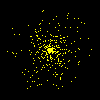Expansion and Contraction of the Universe.

The Aspect Experiments (also view discussion)
Below: The polarization correlation (coincidence count rate) of visible photons emitted in a two-photon-calcium-atomic cascade from a state pumped using two lasers are measured using independently rotated polarizers. The exactly-correlated photons are both transmitted when the mutually aligned polarizers are aligned sufficiently with each photon’s optical axis to cause a coincidence count. They are both blocked when the mutually aligned polarizers are aligned sufficiently perpendicular to each photon’s optical axis to cause the absence of a coincidence count.